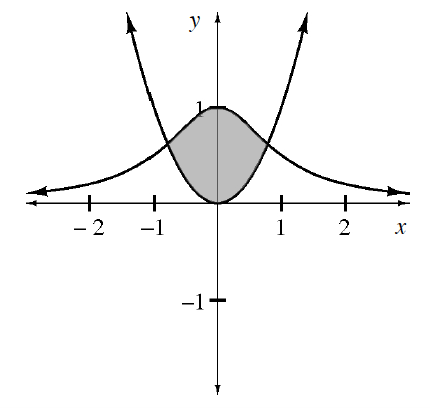### Home > APCALC > Chapter 6 > Lesson 6.3.2 > Problem6-98

6-98.

Calculate the area (shown in the diagram) between the graphs of $y=\frac{1}{x^2+1}$ and $y = x^2$.

Identify which function is on top and which is on the bottom.

$\text{Area between curves}=\int_{A}^{B}((\text{top function})-(\text{bottom function}))dx$

$A =$ left intersection point
$B =$ right intersection point